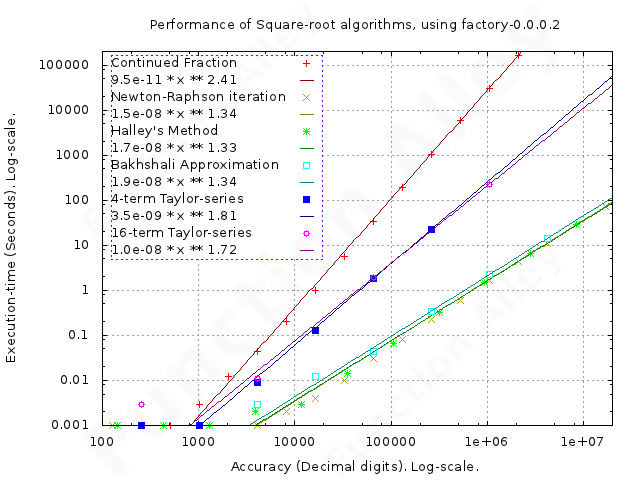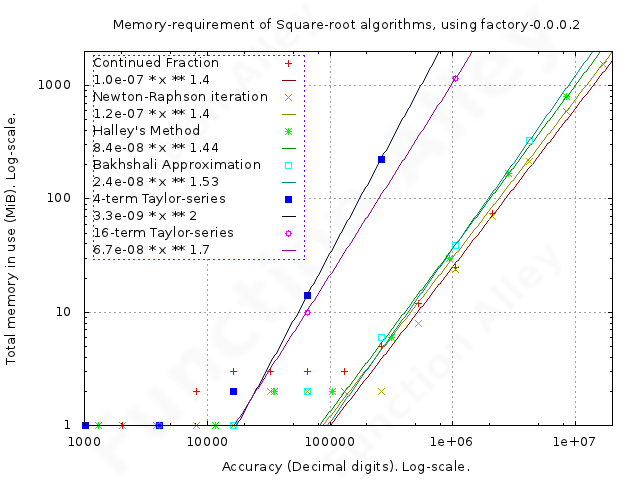# Giving a Hoot about Square-rootsCalculators don't typically facilitate rational arithmetic, merely an uninspiring blend of floating-point & integer (though potentially also in binary or hexadecimal). Perhaps because of this, & their ubiquitous use in imperial units, it's tempting to think of fractional numerical representations as rather quirky & old-fashioned; arithmetic before the Sinclair Executive fixed it. I can't prove that nothing is further from the truth, but I don't need to, because rational arithmetic, in contrast to floating-point, doesn't suffer from rounding-errors, & is therefore the only viable representation, when a precise operation on a real number is required.

Haskell's data-type "Data.Ratio.Ratio", facilitates the exploration of problem-domains which previously would have fallen into the "mañana" category; for example square-roots. The square-root of all but a few privileged numbers (those which can be expressed as a ratio of perfect squares) is actually irrational, but rational arithmetic allows one to approximate their value to an arbitrary precision, far exceeding the most precise standardised floating-point arithmetic. One might naïvely question the burning requirement to estimate the square-root of a number to an arbitrarily precise extent; many of the most effective algorithms to generate Pi to an arbitrary precision, rely on the ability to determine square-roots to a similar precision (e.g. Ramanujan's formula, the Chudnovsky brothers' formula, the Borwein brothers' formula & the Brent-Salamin algorithm). One might then question why Pi is so interesting, but since this philosophical line could extend indefinitely, I'll end where it starts by saying, it isn't, unless mathematics is.

There are almost as many algorithms by which the square-root of a number can be estimated, as there are reasons to use any of them. Each of them takes an estimate for the required value, & improves it, but the mechanism by which this improvement is achieved, falls broadly into two categories; series-expansions & iterations.

Defining the error as the arithmetic discrepancy between the estimated value of the exact solution, then a convergent algorithm will progressively reduce this error, but since the required result is typically irrational, (& therefore can't be represented precisely as a rational number), the error can never reduce to zero, but merely tends towards zero as the algorithm is applied ad-infinitum. As one approaches this limit, the ratio of successive errors is called the "Rate of convergence", which quantifies the number of terms in a series or iterations, required to improve the current estimate to the required degree.

The rate of convergence may be sub-categorised into orders of convergence. If the ratio of successive errors settles to a constant value, then the series is said to have a linear order of convergence, whereas if successive errors settle to a power of the previous error, it is said to converge "super-linearly". Super-linearly convergent algorithms, in which the error is the square of the previous error are said to have a "quadratic" order of convergence, whereas as those in which the error is the cube of the previous are said to have a "cubic" order of convergence … you get the idea. It's less complicated than my explanation makes it; the linear algorithms add a fixed number of correct digits to the estimate at each step, whereas the super-linear algorithms multiply the number of correct digits by their order of convergence. Of the two broad classifications into which the available algorithms may be categorised, the series-expansions converge linearly with the addition of each successive term, whereas the iterations are typically super-linear. Things get more complicated if one evaluates a linearly convergent series to a fixed number of terms, then uses the resulting improved estimate to re-define iteratively a more rapidly convergent series.This is all pretty standard stuff, & may even be largely correct, but what's missing is an analysis of which algorithm is most efficient in practice. Whilst it's tempting to assume that a higher order of convergence is better, because it sounds like someone resembling one of the Mekon, furrowed their brow & ploughed some serious thought into the algorithm, the thought that went in didn't necessarily address the number of steps required to implement the algorithm. To redress this, I've gathered a motley selection of algorithms, implemented them in Haskell (any conclusion drawn from this effort, must account for my limited capabilities), using its Rational type-class, & measured the performance.

Square-root algorithms implemented in package "factory".
Algorithm Order of Convergence Notes
Continued Fraction Linear
Newton-Raphson iteration Quadratic AKA the Babylonian or Heron's method.
Bakhshali Approximation Cubic
Halley's Method Quartic
Taylor-series Linear Converges super-linearly, with an order equal to the number of terms, when applied iteratively.

## Testing

• My usual test-platform was used.

• Though the algorithms were implemented to accept any Real operand, all tests were actually performed arbitrarily on the integer "2". Subsequent testing suggests that whilst this choice makes little difference to performance, the representation of some rational numbers can be very large (irrespective of whether they represent a number of large magnitude), & this would affect the memory-requirement.

## Results

I've fitted polynomials to the data for each algorithm, so that one can estimate their complexity-class. Because of the log-log plot, these polynomials appear as straight lines.• From this one can see that Newton-Raphson iteration, the Bakhshali Approximation & Halley's Method have similar performance.

• The Taylor-series, evaluated rather arbitrarily to both 4 & 16 terms & then applied iteratively to yield quartic convergence & order-16 convergence, perform similarly, but uncompetitively. It seems reasonable to conclude that it is also uncompetitive when evaluated to an arbitrary number of terms.

Performance is sensitive to the speed at which lists of Rational numbers can be added, & significant gains were made after replacing use of the standard polymorphic function "Data.List.sum" (the implementation of which results in lazily folding cross-multiplication over the list), with a function specialised for Rational numbers (which calculates the GHC.Real.lcm of the denominators in the list, then scales the numerators to this common denominator, & adds them). This is effective for the short lists of numbers encountered here, but it has the potential disadvantage, that it blocks garbage-collection of the whole list, until the final sum has been determined. Should the list be longer, or contain much larger rational numbers, then this issue will raise its ugly head. I had hoped that further performance-gains could be achieved, since in any one iteration, one can evaluate all the terms of the series in parallel. Regrettably, this doesn't seem to be an effective strategy on the two-core hardware on which the testing was conducted.

Performance of all the iteration-algorithms, was greatly improved by the elimination of unquantifiable accidental excess precision in the initial Rational estimate, on which they operate. It might be counter-intuitive that excess precision could be problematic, but it is when it results in gross inefficiency. One has to start the iteration with an initial estimate, & that estimate must guarantee a minimum level of accuracy, in order that one may determine the minimum number of iterations required to improve it to the required accuracy. In any specific example, the accuracy of this initial estimate may, by chance, exceed expectations, & when precisely converted to Rational form, may then be burdened by an unfeasibly large numerator & denominator, making arithmetic operations inefficient. This inefficiency can be reduced, if one can find a Rational number of similar magnitude, which has sufficient accuracy, but has a significantly simpler Rational representation. For this purpose, the Haskell-function "Data.Ratio.approxRational" was used.

• Finally, the implementation using Continued fractions, is not only slower than the others at any tested precision-requirement, but given the apparent time-complexity, one may reasonably conclude that it is also the worst at all greater precision-requirements.The memory-requirement, is a second factor in the suitability of these algorithms. In this respect Continued Fractions joined the most competitive performers; Newton-Raphson iteration, the Bakhshali Approximation & Halley's Method; with the two instances of Taylor-series bringing up the rear (though with so few samples that it's rather difficult to draw any reliable conclusions about how much worse they were).

## Conclusions

Algorithms with a low order of convergence, have an unexpected benefit, since the lower it is, the smaller is the likely accuracy-overshoot, & the smaller is the wasted effort for the task in-hand. In the case of the Taylor-series; evaluated to 16 terms, then iteratively re-composed using this improved estimate, to form a more rapidly convergent series; convergence is so rapid, that in very few iterations the available memory is exhausted, leaving little choice in the resultant accuracy, & a correspondingly high probability that this choice is unsatisfactory.

In this implementation, Newton-Raphson iteration is marginally the best:

• it was amongst the fastest, & its time-complexity suggested the it would remain so at higher accuracies;
• it's memory-requirement was lower than competitors of similar speed;
• it has a very manageable quadratic order of convergence;
• it was the simplest to implement.